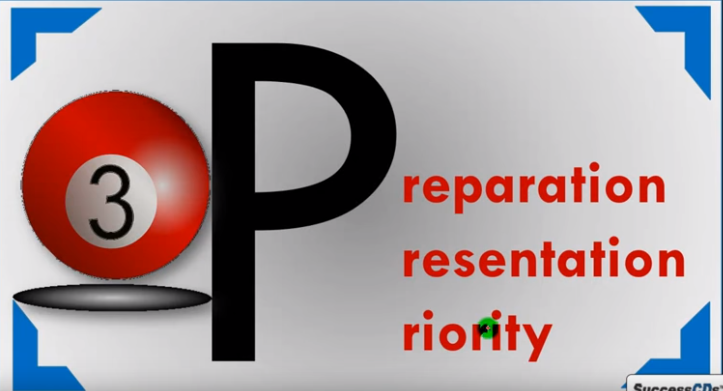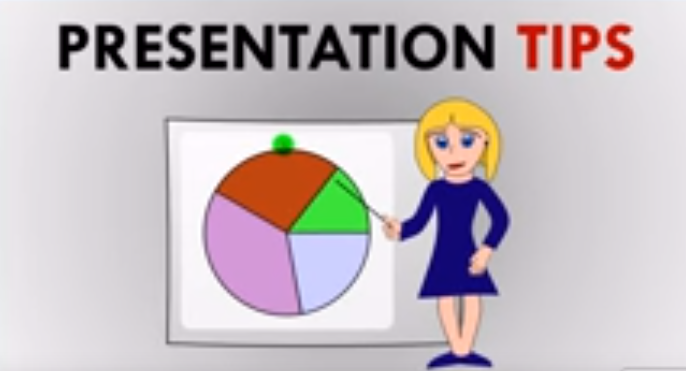Home >> Class 10 >> Science >>

# How to Score 95% in Class 10 Science PaperHere, we are going to discuss how to score 95% marks in CBSE Class 10 Science examination. If you are not happy with your marks or want to score maximum marks, you should follow these instructions.The topics we are going to cover here are 3Ps which are as follows:

1. Preparation
2. Presentation
3. Priority

### Firstly we are going to discuss how to attempt chemistry.

To attempt chemistry we need to follow the instructions mentioned below-

### Preparation Tips

1. The most important thing that we should do is that we should always use examples to explain definitions.

For example: If the question is based on type of reactions, pH, and acid and base. To score good marks we need to explain it with the help of examples. Like firstly, we need to write about the types of reactions including what is reaction and how many types of reactions are there. Then we need to explain each type in detail by using suitable examples. The important questions include redox reaction and displacement reaction. In that cases, we need to follow the instructions written above and we need to write chemical reaction for this. As chemical reactions makes our paper more attractive and show that the student has enough knowledge about this, it will help you score full marks in every question.
pH: For this we need to learn about pH including the pH of water. If they ask what is pH?, then you need to write definition of pH first and then to get good score, you need to write something in addition like pH of water.
Acid and bases: In this case, firstly you need to learn about what is acid and base. Then you need to write examples of both.2. You need to give extra revision time to derivations, formulas (mirror formulas), diagrams (ray diagrams), chemical equations, nomenclature (baking soda), and chemical name.
3. Then you need to go through previous year question papers especially for weaker section.

For example, your chemistry portion is weak and you want to score high marks in chemistry. For this, what you need to do is that you should go through previous year question papers. In previous year question papers you need to focus on type of questions and their scoring (like 2 marks or 5 marks). Most of the times, previous year questions are repeated. By doing this you can score high mark in your weaker section also. Similarly with physics, if your physics is weak, you need to go through previous year question paper of physics.
You should make notes for revision for biology and chemistry. You can also make points for particular questions or important questions like for Mendeleev’s periodic table. If you do not want to make notes, you can use the book directly also. For this, you need to use marker or highlighter to highlight the important lines or words in your book so that while revising, you can easily go through important topics.

4. You should not restrict yourselves to CBSE Class 10 NCERT book .

Sometimes, students face difficulty while learning from NCERT book. In that case, you need to follow other books or notes from which you can learn easily. You can also refer to online study material for that particular question.

### Presentation Tips1. The first presentation tip is that you need to write in points especially in five marks or 3 marks questions.

To exemplify, let us take an example:
A resource is any physical material constituting part of earth that people need and value. Natural materials become resources when humans value them. The uses and values of resources change from culture to culture and from time to time.  To make your paper more attractive, you need to write it as follows:

• A resource is any physical material constituting part of earth that people need and value.
• Natural materials become resources when humans value them.
• The uses and values of resources change from culture to culture and from time to time.

If you will write the answer in this format like making points and highlighting the important words (which can be done by using pencil), you will definitely score full marks.

2. Make completely labeled diagrams wherever possible as it is very important to get full marks in Science.

For example, if you make the diagram of human eye, you need to label the diagram well like mentioning where is pupil, iris, cornea, retina, etc. It will help you get 95+ marks in science. You need to follow the same instructions for ray diagrams also. You can also make block diagrams wherever required.

3. You should highlight the important things by underlining with pencil.

For example, if you write chemical reactions in your paper, you can make a box in which the chemical reactions can be written. You can also highlight the important words with pencil in case of derivation or its results. You can also highlight the headings and sub headings with pencil. You can also make a box for results of numericals so that the result is highlighted and examiner can directly focus on your result or answer.

### Priority tips

In this we will discuss which question should be attempted first and which one at the end.As you all know that the CBSE Class 10 Science Examination Question paper is divided into 2 parts:

• Section A is a theoretical part
• Section B is a part in which practical questions are asked.

Let us discuss the sequence in which the questions should be attempted-
1. We firstly need to attempt 1 marks question. There are only two questions having 1 mark. For these questions we should not devote more than 5 minutes.
2. Then 5 marks questions which are the most lengthy questions should be attempted. The total number of questions carrying 5 marks are 6. For these questions, we need to spend only 60 minutes.
3. Then you need to attempt 2 marks question. The total number of question carrying 2 marks is 3. For these questions, you should not spend more than 15 minutes.
4. Then you need to attempt 3 marks questions. The total number of questions carrying 3 marks is 10. You should spend only 55 minutes for these questions.
For this section, you need to spend a total of 2 hours and 15 minutes.

Section B

Section B of the CBSE Class 10 Science question paper consists of  practical questions.

In section B, firstly you need to attempt 2 marks question. Total number of questions carrying 2 marks each is 6.

For this section, you need to give only 30 minutes.

Then the rest of 15 minutes should be spent for revision purpose.

This revision is very necessary as it is helpful to go through the whole paper and if in any case you forget to attempt the question, you can easily write it while revising.

In this way you can maximize your score in CBSE Class 10 Science Examination.